## Example Questions

### Example Question #51 : How To Find Synthesis Of Data In Physics

Metal wires are often used to conduct electricity. The electricity that flows through a wire is measured as "current." Therefore, a wire with an electric current is called a current carrying wire.

Current carrying wires also have a tendency to produce magnetic fields that circulate around them. The strength of that magnetic field is determined by the following equation whereis the magnetic field strength (Teslas),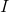is the electric current (Amps),is the distance (meters) of an object to the current carrying wire.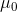is a constant.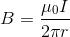The magnitude of a current in a wire,, is affected by several factors. One of the most important of these factors is the resistivity of the substance used to make that wire. Resistivity, often measured in microOhms, is a measure of how much a substance resists the passage of an electrical current through it. Copper, for example, has a low resistivity and therefore can easily hold large currents. Wood, on the other hand has extremely high resistivity and therefore does not hold currents well.

Which of the following substances, paired with their resistivities, would we want to use to make a wire that would produce the strongest magnetic field possible when attaching it to a battery?

Iridium: resistivity = 4.7 microOhms

Platinum: resistivity = 10.6 microOhms

Gold: resistivity = 2.2 microOhms

Silver: resistivity = 1.55 microOhms

Osmium: resistivity = 8.12 microOhms

Silver: resistivity = 1.55 microOhms

Explanation:

The correct answer is silver. Based on the passage, we can discern that the lower a substance's resistivity, the more current that it can hold. With current on in the numerator of the given equation, we want the current to be high so that a high magnetic field can result. Therefore, a wire with the lowest resistivity (and therefore the greatest current) would produce the strongest magnetic field.

### Example Question #52 : How To Find Synthesis Of Data In Physics

Sometimes scientists need to scale down experiments because collecting full-scale data may not be practical or possible. Most of the time, the data collected during a small scale-experiment is an accurate indicator of full-scale results. For example, research of craters on the Earth’s surface has indicated that the Earth has experienced a history of massive meteor collisions. It has been hypothesized that, amongst other things, these impacting meteorites caused the Earth’s oceans to vaporize. The detrimental effects associated with meteor collisions would have made the planet to be uninhabitable to humans. Due to these adverse effects, an experiment that could produce meteor-sized craters would not be possible in a full-scale setting.

Researchers have developed small-scale experiments that are exemplary of Newton’s three laws of motion. Newton’s first law states that an object in motion will stay in motion unless it is acted on by another force. This phenomenon is known as inertia. The second law comes in the form of an equation that calculates how much force is exerted on two colliding objects with respect to mass and acceleration. In other words force is equal to the mass of an object multiplied by its acceleration. The third law states that for every action there is an equal and opposite reaction. In other words, forces always occur in pairs. A counter force that is equal in magnitude and opposite in direction compliments each force in a particular direction. For example, if you push downwards on a table then the table, in theory, pushes upwards with the same force.

In a particular small-scale exercise, students were asked to design an experiment in which two “meteorites” would be dropped into a medium of sand and the subsequent crater diameters would be measured. Afterwards, the sand would be leveled and the experiment would be repeated. The students were asked to identify all of the factors that could affect the formation of the craters. This was done in order to ensure that each experiment was testing one independent and one dependent variable at a time. Otherwise, it would be unclear which variable is causing the observed effect. Possible variables that could alter the outcome of the experiment included but were not limited to the following: height of the fall, speed of the “meteorite,” angle of impact, and the mass of meteorite.

Consider an experiment that seeks to identify the mass of the meteorite analog. All other variables would have to remain constant in order to ensure accurate results.  In order to do this, the height of the fall was set to one meter. The object was placed on top of a vertical meter stick. In order to keep the acceleration and height of the drop constant, it was rolled off of the stick instead of being pushed or simply dropped. Last, a protractor was used to keep the meter stick at a ninety-degree angle. In the procedure, a marble and a golf ball were used as “meteorites” of different masses.

The results of the experiment were recorded in the table and figure provided.

 Crater Diameter (cm) Trial Number Marble (mass = 4g) Golf ball (mass = 46g) 1 4.5 7.75 2 4.5 6 3 4 5.5 4 3 6 5 3 6.5 6 3.5 7 7 3 5 8 3 5 9 3 7 10 3.5 6 Average Average 3.5 cm 6.175 cm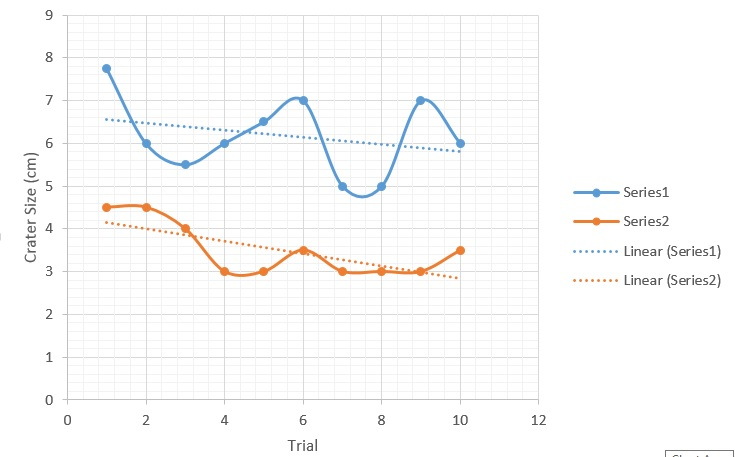Which the following energies is being exhibited by the marble when it is at rest on top of the meter stick?

Potential energy

Kinetic energy

Chemical energy

Mechanical energy

Potential energy

Explanation:

Energy is the ability to do work. When an object is in motion, it is called kinetic energy. When an object is static it said to have potential energy. It can also be described as stored energy. An example of this is a stopped car or a roller coaster sitting at the top of the ramp before descent.

### Example Question #53 : How To Find Synthesis Of Data In Physics

Sometimes scientists need to scale down experiments because collecting full-scale data may not be practical or possible. Most of the time, the data collected during a small scale-experiment is an accurate indicator of full-scale results. For example, research of craters on the Earth’s surface has indicated that the Earth has experienced a history of massive meteor collisions. It has been hypothesized that, amongst other things, these impacting meteorites caused the Earth’s oceans to vaporize. The detrimental effects associated with meteor collisions would have made the planet to be uninhabitable to humans. Due to these adverse effects, an experiment that could produce meteor-sized craters would not be possible in a full-scale setting.

Researchers have developed small-scale experiments that are exemplary of Newton’s three laws of motion. Newton’s first law states that an object in motion will stay in motion unless it is acted on by another force. This phenomenon is known as inertia. The second law comes in the form of an equation that calculates how much force is exerted on two colliding objects with respect to mass and acceleration. In other words force is equal to the mass of an object multiplied by its acceleration. The third law states that for every action there is an equal and opposite reaction. In other words, forces always occur in pairs. A counter force that is equal in magnitude and opposite in direction compliments each force in a particular direction. For example, if you push downwards on a table then the table, in theory, pushes upwards with the same force.

In a particular small-scale exercise, students were asked to design an experiment in which two “meteorites” would be dropped into a medium of sand and the subsequent crater diameters would be measured. Afterwards, the sand would be leveled and the experiment would be repeated. The students were asked to identify all of the factors that could affect the formation of the craters. This was done in order to ensure that each experiment was testing one independent and one dependent variable at a time. Otherwise, it would be unclear which variable is causing the observed effect. Possible variables that could alter the outcome of the experiment included but were not limited to the following: height of the fall, speed of the “meteorite,” angle of impact, and the mass of meteorite.

Consider an experiment that seeks to identify the mass of the meteorite analog. All other variables would have to remain constant in order to ensure accurate results.  In order to do this, the height of the fall was set to one meter. The object was placed on top of a vertical meter stick. In order to keep the acceleration and height of the drop constant, it was rolled off of the stick instead of being pushed or simply dropped. Last, a protractor was used to keep the meter stick at a ninety-degree angle. In the procedure, a marble and a golf ball were used as “meteorites” of different masses.

The results of the experiment were recorded in the table and figure provided.

 Crater Diameter (cm) Trial Number Marble (mass = 4g) Golf ball (mass = 46g) 1 4.5 7.75 2 4.5 6 3 4 5.5 4 3 6 5 3 6.5 6 3.5 7 7 3 5 8 3 5 9 3 7 10 3.5 6 Average Average 3.5 cm 6.175 cmThe equation for Kinetic energy (energy in motion) is as follows: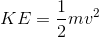In this equation, kinetic energy equals one half of the mass of the object times its velocity squared. Based on this information, identify which factor has the greatest effect on kinetic energy?

Volume

Velocity

Mass

None of these

Velocity

Explanation:

We are given the following equation:Given the equation, it is clear that velocity plays a larger role in kinetic energy than mass. This is due to the exponential relationship related to the velocity while mass only possesses a linear relationship.

### Example Question #54 : How To Find Synthesis Of Data In Physics

Sometimes scientists need to scale down experiments because collecting full-scale data may not be practical or possible. Most of the time, the data collected during a small scale-experiment is an accurate indicator of full-scale results. For example, research of craters on the Earth’s surface has indicated that the Earth has experienced a history of massive meteor collisions. It has been hypothesized that, amongst other things, these impacting meteorites caused the Earth’s oceans to vaporize. The detrimental effects associated with meteor collisions would have made the planet to be uninhabitable to humans. Due to these adverse effects, an experiment that could produce meteor-sized craters would not be possible in a full-scale setting.

Researchers have developed small-scale experiments that are exemplary of Newton’s three laws of motion. Newton’s first law states that an object in motion will stay in motion unless it is acted on by another force. This phenomenon is known as inertia. The second law comes in the form of an equation that calculates how much force is exerted on two colliding objects with respect to mass and acceleration. In other words force is equal to the mass of an object multiplied by its acceleration. The third law states that for every action there is an equal and opposite reaction. In other words, forces always occur in pairs. A counter force that is equal in magnitude and opposite in direction compliments each force in a particular direction. For example, if you push downwards on a table then the table, in theory, pushes upwards with the same force.

In a particular small-scale exercise, students were asked to design an experiment in which two “meteorites” would be dropped into a medium of sand and the subsequent crater diameters would be measured. Afterwards, the sand would be leveled and the experiment would be repeated. The students were asked to identify all of the factors that could affect the formation of the craters. This was done in order to ensure that each experiment was testing one independent and one dependent variable at a time. Otherwise, it would be unclear which variable is causing the observed effect. Possible variables that could alter the outcome of the experiment included but were not limited to the following: height of the fall, speed of the “meteorite,” angle of impact, and the mass of meteorite.

Consider an experiment that seeks to identify the mass of the meteorite analog. All other variables would have to remain constant in order to ensure accurate results.  In order to do this, the height of the fall was set to one meter. The object was placed on top of a vertical meter stick. In order to keep the acceleration and height of the drop constant, it was rolled off of the stick instead of being pushed or simply dropped. Last, a protractor was used to keep the meter stick at a ninety-degree angle. In the procedure, a marble and a golf ball were used as “meteorites” of different masses.

The results of the experiment were recorded in the table and figure provided.

 Crater Diameter (cm) Trial Number Marble (mass = 4g) Golf ball (mass = 46g) 1 4.5 7.75 2 4.5 6 3 4 5.5 4 3 6 5 3 6.5 6 3.5 7 7 3 5 8 3 5 9 3 7 10 3.5 6 Average Average 3.5 cm 6.175 cmUsing your understanding of kinetic energy and the information presented in the graph—where Series 1 is a golf ball and Series 2 is a marble—which of the following represents the most valid conclusion?

Remember:According to the results, there is an increasing exponential correlation between mass and crater size (i.e. as the mass increases, the crater diameter increases).

According to the results, there is an increasing linear correlation between mass and crater size (i.e. as the mass increases, the crater diameter increases).

According to the results, there is a decreasing linear correlation between mass and crater size (i.e. as the mass increases, the crater diameter decreases).

According to the results, is a decreasing exponential correlation between mass and crater size (i.e. as the mass increases, the crater diameter decreases).

According to the results, there is an increasing linear correlation between mass and crater size (i.e. as the mass increases, the crater diameter increases).

Explanation:

When looking at the graph, it is clear that there is an increase in crater size as the mass increases. Using the understanding of kinetic energy, we know that mass has a linear correlation with force.

Assume the following velocity: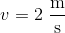At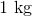, the force would be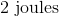.

At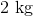, the force would be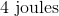.

At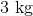, the force would be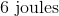.

At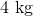, the force would be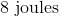.

For every one-unit increase in mass, there is a two-unit increase in force. This is what is known as a linear correlation.

### Example Question #55 : How To Find Synthesis Of Data In Physics

The cells of multicellular organisms must be linked to each other through special structures called cellular junctions. A gap junction is a type of cellular junction, which allows for the passage of small molecules for fast coordination between cells. For example, cardiac cells in the heart and neurons in the brain rely on gap junctions. However, gap junctions are small and there is a limit to how large a molecule can be in order for it to pass through the gap junction.

An experiment is designed to see how large molecules can be to pass through a gap junction. A single layer of cells is grown in a petri dish. A single cell is then injected with a dye labeled with a fluorescent molecule. Researchers observe the following: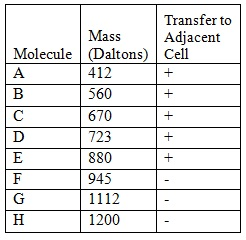Another scientist repeats the study; however she obtains the following results: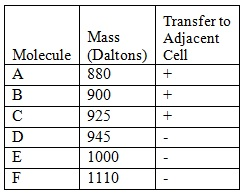What does her data suggest? Pick the most comprehensive conclusion.

Gap junctions allow molecules 925 Daltons or less to pass through.

Gap junctions allow molecules 925 Daltons or greater to pass through.

Gap junctions allow molecules 925 Daltons or less to pass through but they do not allow molecules 1000 Daltons or greater to pass through.

Gap junctions allow molecules 925 Daltons or smaller to pass through but they do not allow molecules 945 Daltons or greater to pass through.

Gap junctions allow molecules 925 Daltons or smaller to pass through but they do not allow molecules 945 Daltons or greater to pass through.

Explanation:

The correct answer is the more comprehensive statement, "Gap junctions allow molecules 925 Daltons or smaller to pass through but they do not allow molecules 945 Daltons or greater to pass through." The other statements may be correct but they do not define the more specific limits of the gap junctions.

### Example Question #56 : How To Find Synthesis Of Data In Physics

Students are given an assignment to create a circuit from a given set of components. Four different students create circuits in unique manners and the professor measures the resistance of the circuit, the power output of the circuit and the heat given off by each circuit after each circuit was running for a certain amount of time. Circuits with resistors in parallel have a lower total resistance than those that have resistors in series.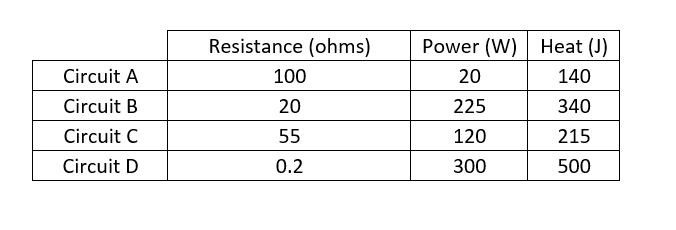One circuit was set up with all of its resistors in parallel. Which circuit is most likely the one with all of its resistors in parallel?

Circuit B

Circuit C

Circuit D

Cannot be determined from the given information

Circuit A

Circuit D

Explanation:

When resistors are placed in series, the overall resistance is increased. On the other hand, when resistors are placed in parallel the overall resistance is decreased. If all circuits are made of the same component and one only had resistors in parallel, this would cause the lowest observed resistance and this is Circuit D.

### Example Question #57 : How To Find Synthesis Of Data In Physics

Students are given an assignment to create a circuit from a given set of components. Four different students create circuits in unique manners and the professor measures the resistance of the circuit, the power output of the circuit and the heat given off by each circuit after each circuit was running for a certain amount of time. Circuits with resistors in parallel have a lower total resistance than those that have resistors in series.Which circuit would be best suited for powering an electric generator?

Cannot be determined from the given information

Circuit C

Circuit A

Circuit B

Circuit D

Cannot be determined from the given information

Explanation:

In a generator, it would be ideal to have a high power output, however there is no information on how heat would affect a generator. For example, if a lot of heat is given off it could cause the generator to overheat and fail. No information is provided on the ideal amount of power to heat ratio for a generator so the answer cannot be determined from the given information.

### Example Question #58 : How To Find Synthesis Of Data In Physics

Scientist 1: Scientist 1 believes that light displays particle behavior. This means that rays of light have their own associated momentum. Furthermore, Scientist 1 does not believe that light will exhibit wave behavior.

Scientist 2: Scientist 2 disagrees with Scientist 1 and believes that light can exhibit wave behavior, but does not display particle behavior. In other words, this scientist believes that light does not have any momentum.

Experiment: To settle their disagreement, the scientists setup the following experiment. The scientist take dark metallic material. This material is attached to pole and the metallic material can spin if it is subjected to a force; similar to a watermill or wind turbine. This setup is then placed outside and exposed to sunlight.

Assuming that sunlight displays particle behavior, what is the best way to design a device to absorb solar energy and turn it into electrical energy?

Use solar cells placed underwater to collect refracted sunlight

A flat solar panel facing the sun

Cannot be determined from the given information

Solar cells placed on the surface of a wind turbine

Solar cells placed in the shade, but with reflected sunlight aimed at the solar cells

Cannot be determined from the given information

Explanation:

It is assumed that light exhibits particle behavior with momentum, but there is no information on how this affects the amount of sunlight absorbed. In addition, there is no information given on how to effectively build a device to absorb sunlight that can be converted into electrical energy so the answer cannot be determined.

### Example Question #121 : Physics

A scientist is exploring the nature of energy and work in two experimental systems.

Experiment 1

She first sets up a system with a ball on an inclined plane, and calculates the potential and kinetic energies of the ball at three positions. She places the ball at position 1, stops the ball after rolling to position 2, and then again allows it to roll to position 3. The measured kinetic and potential energies are shown in the table, along with measurements of the force of friction acting on the ball.

 Ball Position Potential Energy Kinetic Energy Force of Friction 1 150 kJ X kJ 0 N 2 75 kJ 75 kJ 0 N 3 X kJ 150 kJ 0 N

Experiment 2

The same scientist then sets up another experiment with the same ball, again at three positions. The ball is provided a slight push, and then allowed to roll down three levels without any additional external input of energy.

She uses the following formulae to calculate the energy levels of the ball:

Potential Energy = Mass of the Ball x Acceleration Due to Gravity x Height of the Ball

Kinetic Energy = (1/2) x Mass of the Ball x (Velocity of the Ball)2

She also measures the internal energy of the ball, a value that she defines as the amount of energy contained in the motion of the molecules that make up the matter of the ball.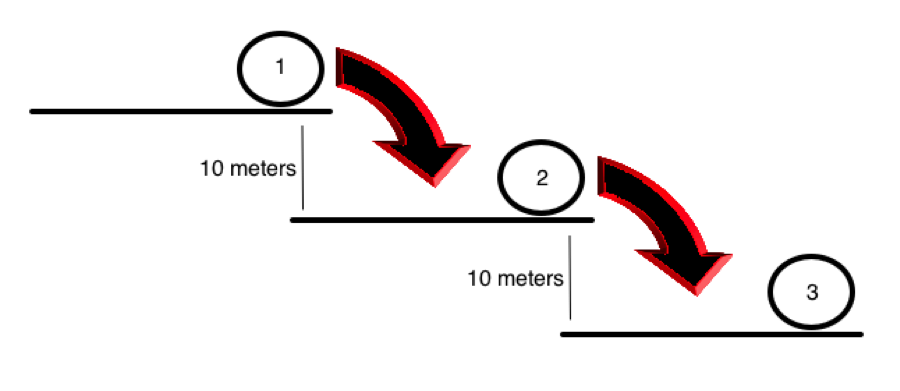Position Potential Energy Velocity Internal Energy 1 A 1 m/s 5 kJ 2 B 5.47 m/s 6 kJ 3 C 10.94 m/s 7 kJ

In Experiment 2, which of the following is most likely true?

Point 1 will have the greatest potential energy and the least kinetic energy.

Point 2 will have the greatest potential energy and the least kinetic energy.

Point 1 will have the greatest kinetic energy and the least potential energy.

Point 3 will have the greatest potential energy and the least kinetic energy.

Point 1 will have the greatest potential energy and the least kinetic energy.

Explanation:

In the passage, we are given the formulae for potential and kinetic energies. From these, we can see that potential energy is dependent on the height of the object in question, while kinetic energy is dependent on the object's velocity. Therefore, we would expect the object will have the greatest potential energy at its highest point, or point 1.

### Example Question #60 : How To Find Synthesis Of Data In Physics

A scientist is exploring the nature of energy and work in two experimental systems.

Experiment 1

She first sets up a system with a ball on an inclined plane, and calculates the potential and kinetic energies of the ball at three positions. She places the ball at position 1, stops the ball after rolling to position 2, and then again allows it to roll to position 3. The measured kinetic and potential energies are shown in the table, along with measurements of the force of friction acting on the ball.

 Ball Position Potential Energy Kinetic Energy Force of Friction 1 150 kJ X kJ 0 N 2 75 kJ 75 kJ 0 N 3 X kJ 150 kJ 0 N

Experiment 2

The same scientist then sets up another experiment with the same ball, again at three positions. The ball is provided a slight push, and then allowed to roll down three levels without any additional external input of energy.

She uses the following formulae to calculate the energy levels of the ball:

Potential Energy = Mass of the Ball x Acceleration Due to Gravity x Height of the Ball

Kinetic Energy = (1/2) x Mass of the Ball x (Velocity of the Ball)2

She also measures the internal energy of the ball, a value that she defines as the amount of energy contained in the motion of the molecules that make up the matter of the ball.Position Potential Energy Velocity Internal Energy 1 A 1 m/s 5 kJ 2 B 5.47 m/s 6 kJ 3 C 10.94 m/s 7 kJ

The scientist recalls that, roughly, the kinetic energy of molecules in matter corresponds to the temperature of that piece of matter when measured at the macroscopic scale. Which of the following is most likely true of the ball in Experiment 2?

It has a slightly higher temperature at position 1 than position 2.

It has a slightly higher temperature of position 3 than position 2.

It has a slightly lower temperature at position 3 than position 2.

It has exactly the same temperature throughout the experiment.